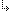- 4.37.2.5. -4. Standard Units4.37. Graph - Graph unit4.37.2. Graph Unit Procedures and Functions4.37.2.5. Circle procedure

## 4.37.2.5. Circle procedure

Targets: MS-DOS, Win32

Graph Unit

Draws a circle in the current color set by SetColor, using (X,Y) as the center point.

Declaration:
```procedure Circle(X,Y: Longint; Radius: DWORD);
procedure Circle(X,Y: Longint; Radius, Color: DWORD);```
Remarks:
Draws a circle in the current color set by SetColor. Each graphics mode has an aspect ratio used by Circle.

- 4.37.2.5. -